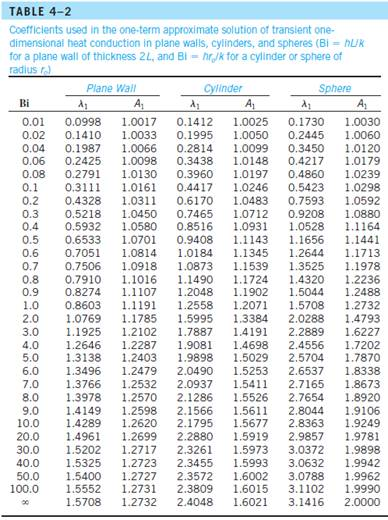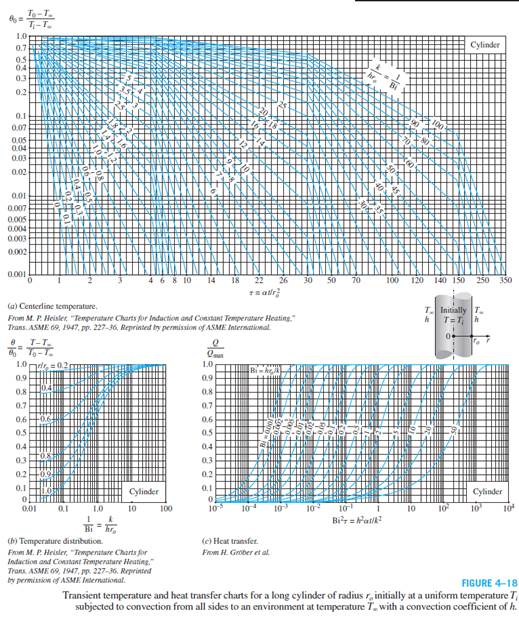Create an Account

Home / Questions / A long Pyroceram rod

A long Pyroceram rod

A long Pyroceram rod (ρ = 2600 kg/m3, cp = 808 J/kg·K, k = 3.98 W/m·K, and α = 1.89 × 10–6 m2 /s) with diameter of 10 mm has an initial uniform temperature of 1000°C. The Pyroceram rod is allowed to cool in ambient temperature of 25°C and convection heat transfer coefficient of 80 W/m2 ·K. If the Pyroceram rod is allowed to cool for 3 minutes, determine the temperature at the center of the rod using

(a) Table 4–2 and(b) the Heisler chart (Figure 4–18).Jul 24 2020 View more View LessSubscribe To Get Solution